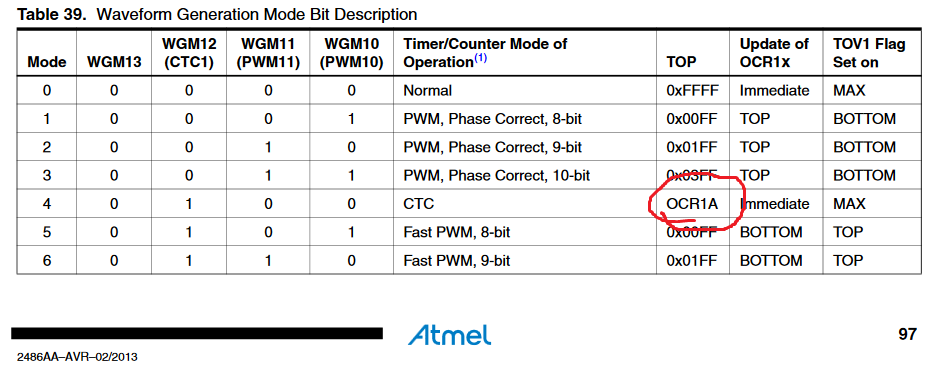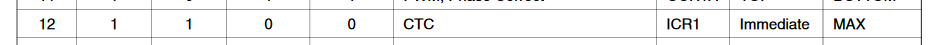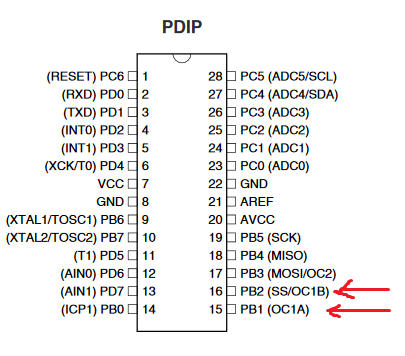## How to use TIMER1_COMPB_vect on AtMega8.

6 posts / 0 new
Author
Message

Hi there!

First of all, I'm sorry if you will find mistakes in my text. English is not my native language.

If you have a simple code, how to work with Timer1_COMPB_vect on AtMega8, I will be a very grateful.

So.

I am trying to understand how to work with Timer1_COMPB_vect and I wrote the code, but my code doesn't work.

My code:

```ISR (TIMER1_COMPB_vect){
cli ();
PORTD ^= (1 << PC0);
//Example code
sei ();
}

int main (void){

DDRC |= (1 << DDC0);
PORTC |= (1 << PC0);

TCNT1 = 0x0000; // Initial value is zero
TCCR1B |= (1 << CS12) | (1 << CS10); // clk / 1024. 8*10^6/1024 = 7812 tics/sec.
OCR1B = 7812; // 1 sec.
TIMSK |= (1 << OCR1B); // Interrup of OCR1B.

sei (); // Global interrrupt.

while (true){}

return 0;

}```

Thanks!

Hello, Feni, and welcome to AVR Freaks -

How do you determine that it does not work?

As a side note, which should not effect how it works, the sei() and cli() in the interrupt at totally not necessary. Those two operations are automatically done by hardware on every ISR call and every ISR return.

Jim

Until Black Lives Matter, we do not have "All Lives Matter"!

Last Edited: Mon. Apr 9, 2018 - 07:14 PM

Please post a complete test program.  Tell your AVR model and clock speed.  Tell your language, brand of toolchain and version.  Tell compile options, and build results.  Are there any warnings?Show your schematic and describe what is connected.

What do you expect to happen?  What >>is<< happening?  How are you testing?

Have you looked at the timer-related articles in the Tutorials forum?

Feni wrote:
TIMSK |= (1 << OCR1B); // Interrup of OCR1B.

Surely that cannot be correct, as OCR1B really isn't anything, but is sometimes used as a shorthand for the register pair OCR1BL and OCR1BH at addresses 0x48 and 0x49. Did you mean OCIE1B?

Feni wrote:
ISR (TIMER1_COMPB_vect){ cli (); PORTD ^= (1 << PC0); //Example code sei (); }

Read the datasheet section on interrupt handling again.  The cli() isn't needed as

When an interrupt occurs, the Global Interrupt Enable I-bit is cleared and all interrupts
are disabled.

and

The I-bit is
automatically set when a Return from Interrupt instruction – RETI – is executed.

You can put lipstick on a pig, but it is still a pig.

I've never met a pig I didn't like, as long as you have some salt and pepper.

```ISR (TIMER1_COMPB_vect){
cli ();
PORTD ^= (1 << PC0);
//Example code
sei ();
}```

If you want an interrupt every second then you have to clear the timer in ISR.

```ISR (TIMER1_COMPB_vect){
PORTD ^= (1 << PC0);
TCNT1 = 0;
}```

Edit:

This applies for the original code #1 where nonpwm mode is used.

Last Edited: Tue. Apr 10, 2018 - 09:01 AM

Tell your AVR model and clock speed.

I use AtMega8,  clock speed is 8MHz.

Tell your language, brand of toolchain and version.

I use Atmel Studio 7 in English version

How are you testing?

I'm testing my progects in Proteus 8.6

What do you expect to happen?

Ok. If I write code for TIMER1_COMPA_vect, code works perfectly, but if I change this for  TIMER1_COMPB_vect, it doen't not work.

Code for TIMER1_COMPA_vect:

```ISR(TIMER1_COMPA_vect){
PORTC ^= (1 << PC0);
}

int main (void){
PORTC |= (1 << PC0);
DDRC |= (1 << DDC0);

TCNT1 = 0x0000; // 16-bit timer-counter1. Initial value is zero.
TCCR1B |= (1 << CS12); // clk/256
TCCR1B |= (1 << WGM12); // CTC
TIMSK |= (1 << OCIE1A);

OCR1AH = 0x7F;
OCR1AL = 0xFF; // 32767

sei ();

while (true){}

return 0;

}```

For TIMER1_COMPB_vect doen't works and I can't find a problem.

```ISR (TIMER1_COMPB_vect){
PORTC ^= (1 << PC0);
}

int main (void){
PORTC |= (1 << PC0);
DDRC |= (1 << DDC0);

TCNT1 = 0x0000; // 16-bit timer-counter1. Initial value is zero.
TCCR1B |= (1 << CS12); // clk/256
TCCR1B |= (1 << WGM12); // CTC
TIMSK |= (1 << OCIE1B); // for TIMER1_COMPB_vect

OCR1BH = 0x7F;
OCR1BL = 0xFF; // 32767

sei ();

while (true){}

return 0;

}```

Feni wrote:
doen't works
You still aren't really saying what "doesn't work" means.

Having said that you can't expect B to work exactly like A because of this:You are using mode 4 so "TOP" (the count limit of the timer) is set by the OCR1A register - so in the second program you can't just switch from using OCR1A to OCR1B

I wonder if you might want to explore using mode 12 instead?As you can see, in this mode TOP is set by ICR1 so this would work whether you then used A or B as the output channel.

Also, instead of using PC0 as the output you may want to explore this:The timer can be set to toggle PB1 (channel A) or PB2 (channel B) automatically via the hardware - in this case you souldn't even need the ISR. You just configure the timer and leave it to drive the pin with no further interaction at all (which is the usual use of waveform generation - you only usually resort to toggling pins in ISR when there's some reason you cannot use the pin actually dedicated to the timer)